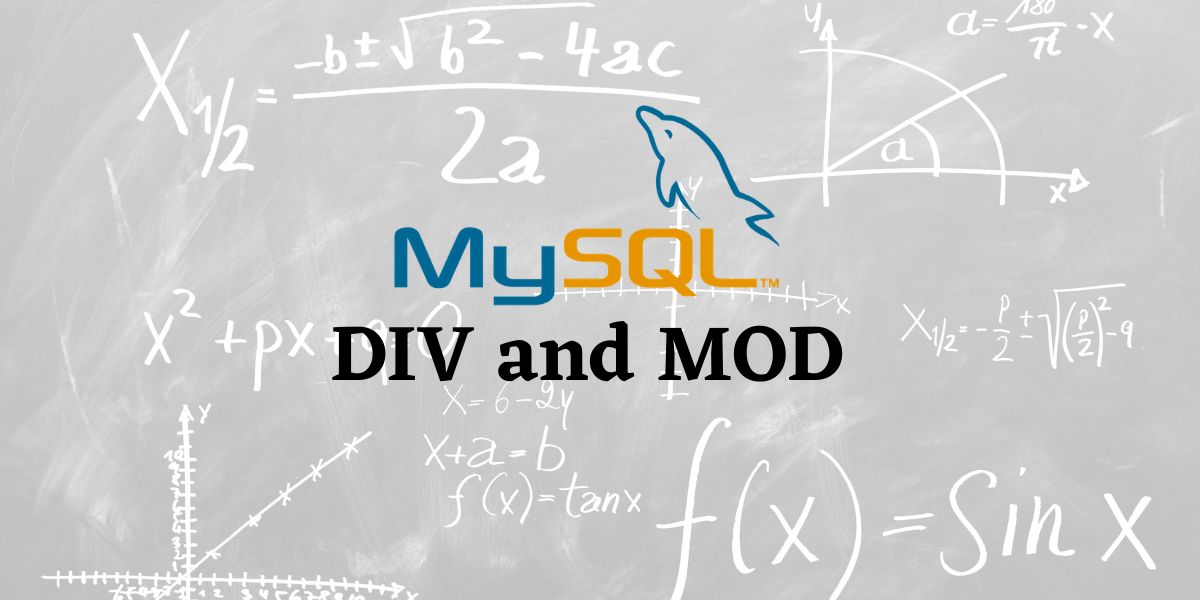# MySQL DIV and MOD – Easy examples to find the quotient and remainder in MySQLIn this tutorial, we will study the MySQL `DIV` and `MOD` functions. As we saw in an earlier article, MySQL provides us with a variety of arithmetic functions. We will explore the `DIV` and `MOD` functions in this one.

• The MySQL `DIV` function performs the integer division of two numbers and returns the quotient. An important point to note here is – integer division. That means, our result is always the integer part in the quotient. We do not get any decimal points in the result.
• The MySQL `MOD` function is used to return the remainder after a number is divided by another number.

Let us now look at their syntax and then their examples.

## Syntax of MySQL DIV

```.wp-block-code {
border: 0;
}

.wp-block-code > span {
display: block;
overflow: auto;
}

.shcb-language {
border: 0;
clip: rect(1px, 1px, 1px, 1px);
-webkit-clip-path: inset(50%);
clip-path: inset(50%);
height: 1px;
margin: -1px;
overflow: hidden;
position: absolute;
width: 1px;
word-wrap: normal;
word-break: normal;
}

.hljs {
box-sizing: border-box;
}

.hljs.shcb-code-table {
display: table;
width: 100%;
}

.hljs.shcb-code-table > .shcb-loc {
color: inherit;
display: table-row;
width: 100%;
}

.hljs.shcb-code-table .shcb-loc > span {
display: table-cell;
}

.wp-block-code code.hljs:not(.shcb-wrap-lines) {
white-space: pre;
}

.wp-block-code code.hljs.shcb-wrap-lines {
white-space: pre-wrap;
}

.hljs.shcb-line-numbers {
border-spacing: 0;
counter-reset: line;
}

.hljs.shcb-line-numbers > .shcb-loc {
counter-increment: line;
}

.hljs.shcb-line-numbers .shcb-loc > span {
}

.hljs.shcb-line-numbers .shcb-loc::before {
border-right: 1px solid #ddd;
content: counter(line);
display: table-cell;
text-align: right;
-webkit-user-select: none;
-moz-user-select: none;
-ms-user-select: none;
user-select: none;
white-space: nowrap;
width: 1%;
}`x DIV y`Code language: SQL (Structured Query Language) (sql)```

Where,

• x is the dividend and,
• y is the divisor.

Note that the `DIV` function and division operator ‘/’ are different. As I said before, `DIV `is for integer division and returns only the integer part of the quotient without the decimal point places. However, the division operator ‘/’ returns decimal points in the result.

## Syntax of MySQL MOD

`MOD` has three syntaxes. You may use either one of them.

``MOD(x, y)`Code language: SQL (Structured Query Language) (sql)`

Or

``x MOD y`Code language: SQL (Structured Query Language) (sql)`

Or

``x % y`Code language: SQL (Structured Query Language) (sql)`

Where,

• x is the dividend and,
• y is the divisor.
• In the third syntax, the % sign is the `MOD` operator.

## Examples of MySQL DIV

Let us look at a few basic examples of `DIV`. Consider the below queries. We will use aliases and the `SELECT` Statement.

``````SELECT 4 DIV 2 AS ‘4/2’;
SELECT 15 DIV 3 AS ‘15/3’;
SELECT 0 DIV 5 AS ‘0/5’;
SELECT 23 DIV 7 AS ‘23/7’;```Code language: SQL (Structured Query Language) (sql)```

And we get our output as,

As you can see, we get the output of the last query as 3, as opposed to 3.2857. To get the quotient with the decimal places, use the division operator ‘/’.

### Difference between MySQL DIV and the division operator.

As I mentioned earlier, `DIV` is for integer division and returns only the integer part of the quotient without the decimal point places. However, the division operator ‘/’ returns decimal points in the result.

Let us see this difference using the below queries.

``````SELECT 23/7 AS ‘23/7’;
SELECT 0/5 AS ‘0/5’;
SELECT 15/3 AS ‘15/3’; ```Code language: SQL (Structured Query Language) (sql)```

These queries are quite similar to what we saw earlier, except that here we are using the division operator. Let us see what we get our output as.

As you can see, the results include at least 4 points of decimal places.

### MySQL DIV With Tables

Consider the below Triangle table.

The table contains the figure IDs of the triangle and the perimeter of each triangle. For this exercise, let us assume that all these triangles are equilateral (all three sides of the triangle are equal in length).

The perimeter of a triangle is the addition of all three sides. Since these triangles are equilateral, their perimeter is 3 times the length of a side. This effectively means that we need to divide the perimeter by 3 to get the measure of the sides of the triangle. So, how about finding the measure of sides of these equilateral triangles? The query for it is,

``SELECT FigureId, Perimeter DIV 3 AS SideOfTriangle FROM Triangle;`Code language: SQL (Structured Query Language) (sql)`

And we get our output as,

Now, how about creating a column for the measure of the side of this equilateral triangle? We do this using,

``````ALTER TABLE Triangle ADD SideOfTriangle int;
UPDATE Triangle SET SideOfTriangle=Perimeter DIV 3;
SELECT * FROM Triangle;```Code language: SQL (Structured Query Language) (sql)```

And the output is,

## Examples of MySQL MOD

Let us look at some basic examples of the MySQL MOD() function. Consider the below queries.

``````SELECT 8 MOD 2 AS Result;
SELECT MOD(15, 6) AS Result;
SELECT 36%10 AS Result;```Code language: SQL (Structured Query Language) (sql)```

And we get the output as,

As you can see, the output is what the remainders we would get if those numbers are divided.

### MySQL MOD With Tables

Let us now see how we can use MySQL `MOD` with tables. Consider the newly updated Triangle table we got after the `DIV` examples. Let us divide the values in the `SideOfTriangle` column by and output the remainder. We do this using the query below.

``SELECT MOD(SideOfTriangle, 5) AS Remainder_After_SideOfTriangle_Divided_by_5 FROM Triangle;`Code language: SQL (Structured Query Language) (sql)`

And we get our output as,

## Conclusion

MySQL `DIV` and `MOD` are very important. I would recommend you to use the division operator instead of `DIV` in most operations. MySQL `MOD` is also very important and finds multiple use cases. I highly recommend you to play around with them and explore them more.